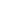# Day 107

## Electric Field Equations

An electric field occupies the space around a charged object. It is created because of the force acting on a test charge, q, by some other source charge, Q.

E = |F|

|q|where q = the value of the test charge in Coulombswhere F = the force acting on the test chargewhere E = the electric field surrounding q

Another equation for the electric field can also be derived by use of Coulombs Law.

Since

F = Eq and F = (kq1q2)/r2, we can set them equal to other:

Eq = (kqQ)/r2Q = the source charge in Coulombs

Removing the test charge, q, from each side, yields.

E = kQ/r2

When do you use E=F/q and when do you use E = kQ/r2?

Use E=F/q when you already know the force on the test charge (due to the source charge).

Use E=kQ/r2 when the force is not known, or the problem wants the electric field strength at some distance from the source charge.

## Electric Field Lines

Field lines can be drawn around a charged object to simulate the strength of the electric field. Positive charges always have field lines that radiate outward and negative charges always have field lines that radiate inward.

The electric field is tangent to the electric field lines at each point.

The number of lines per unit area through a surface perpendicular to the lines is proportional to the strength of the electric field in a given region.

Rules for drawing electric field lines:

1. Lines always begin on positive charges (or infinity) and terminate on negative charges (or infinity)

2. The number of lines leaving a positive charge or terminating on a negative charge is proportional to the magnitude of the charge

3. No two field lines can ever cross each other (not even very far away from the charge!)

### Electric Field Calculation with Multiple Charges

Suppose there are three point charges: -4nC at x = -0.5 m, 5 nC at x = 0 m, and 3 nC at x = 0.8 m. Find the net electric field at point P which is located at x = 2.0 m.

First, determine the field directions from the three individual charges at point P.

The -4 nC charge exhibits an inward field, so for point P it would be directed to the LEFT.

The 5 nC charge exhibits an outward field, so for point P it would be directed to the RIGHT.

The 3 nC charge exhibitis an outward field, so for point P it would be directed to the RIGHT.

Calculate the field strength at point P due to each charge individually.

• E(-4) = kQ/r2 = 9 x 109 Nm2/C2 (-4 x 10-9 C) / (2.5m)2 = 5.76 N/C, LEFT
• E(5) = kQ/r2 = 9 x 109 Nm2/C2 (5 x 10-9 C) / (2.0 m)2 = 11.25 N/C, RIGHT
• E(3) = kQ/r2 = 9 x 109 Nm2/C2 (3 x 10-9 C) / (1.2 m)2= 18.75 N/C, RIGHT
• Enet = 5.76 N/C, LEFT + 11.25 N/C, RIGHT, + 18.75 N/C, RIGHT = 24.24 N/C, RIGHT

Multiple charge problems with the charges on two axes require the use of trigonometry, just as in an electric force problem, since fields and forces are both VECTORS.

(source)